This book is archived and will be removed July 6, 2022. Please use the updated version.

Relativity

# 39 Doppler Effect for Light

### Learning Objectives

By the end of this section, you will be able to:

• Explain the origin of the shift in frequency and wavelength of the observed wavelength when observer and source moved toward or away from each other
• Derive an expression for the relativistic Doppler shift
• Apply the Doppler shift equations to real-world examples

As discussed in the chapter on sound, if a source of sound and a listener are moving farther apart, the listener encounters fewer cycles of a wave in each second, and therefore lower frequency, than if their separation remains constant. For the same reason, the listener detects a higher frequency if the source and listener are getting closer. The resulting Doppler shift in detected frequency occurs for any form of wave. For sound waves, however, the equations for the Doppler shift differ markedly depending on whether it is the source, the observer, or the air, which is moving. Light requires no medium, and the Doppler shift for light traveling in vacuum depends only on the relative speed of the observer and source.

### The Relativistic Doppler Effect

Suppose an observer in S sees light from a source in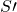moving away at velocity v ((Figure)). The wavelength of the light could be measured within—for example, by using a mirror to set up standing waves and measuring the distance between nodes. These distances are proper lengths withas their rest frame, and change by a factor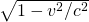when measured in the observer’s frame S, where the ruler measuring the wavelength inis seen as moving.

(a) When a light wave is emitted by a source fixed in the moving inertial frame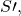the observer in S sees the wavelength measured in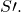to be shorter by a factor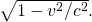(b) Because the observer sees the source moving away within S, the wave pattern reaching the observer in S is also stretched by the factor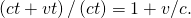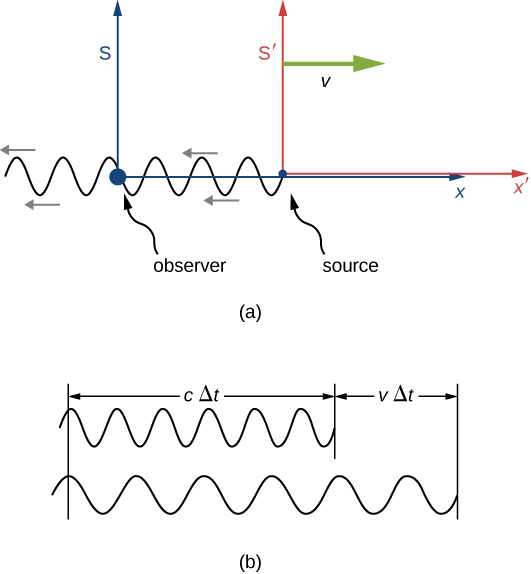If the source were stationary in S, the observer would see a length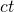of the wave pattern in time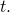But because of the motion ofrelative to S, considered solely within S, the observer sees the wave pattern, and therefore the wavelength, stretched out by a factor of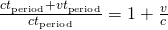as illustrated in (b) of (Figure). The overall increase from both effects gives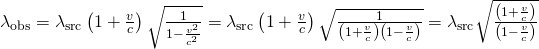where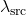is the wavelength of the light seen by the source inand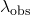is the wavelength that the observer detects within S.

### Red Shifts and Blue Shifts

The observed wavelengthof electromagnetic radiation is longer (called a “red shift”) than that emitted by the source when the source moves away from the observer. Similarly, the wavelength is shorter (called a “blue shift”) when the source moves toward the observer. The amount of change is determined by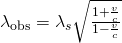where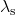is the wavelength in the frame of reference of the source, and v is the relative velocity of the two frames S andThe velocity v is positive for motion away from an observer and negative for motion toward an observer. In terms of source frequency and observed frequency, this equation can be written as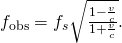Notice that the signs are different from those of the wavelength equation.

Calculating a Doppler Shift Suppose a galaxy is moving away from Earth at a speed 0.825c. It emits radio waves with a wavelength of
0.525 m. What wavelength would we detect on Earth?

Strategy Because the galaxy is moving at a relativistic speed, we must determine the Doppler shift of the radio waves using the relativistic Doppler shift instead of the classical Doppler shift.

Solution

1. Identify the knowns: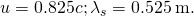2. Identify the unknown: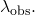3. Express the answer as an equation: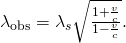4. Do the calculation: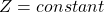Significance Because the galaxy is moving away from Earth, we expect the wavelengths of radiation it emits to be redshifted. The wavelength we calculated is 1.70 m, which is redshifted from the original wavelength of 0.525 m. You will see in Particle Physics and Cosmology that detecting redshifted radiation led to present-day understanding of the origin and evolution of the universe.

Check Your Understanding Suppose a space probe moves away from Earth at a speed 0.350c. It sends a radio-wave message back to Earth at a frequency of 1.50 GHz. At what frequency is the message received on Earth?

We can substitute the data directly into the equation for relativistic Doppler frequency: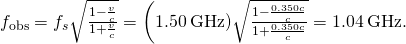The relativistic Doppler effect has applications ranging from Doppler radar storm monitoring to providing information on the motion and distance of stars. We describe some of these applications in the exercises.

### Summary

• An observer of electromagnetic radiation sees relativistic Doppler effects if the source of the radiation is moving relative to the observer. The wavelength of the radiation is longer (called a red shift) than that emitted by the source when the source moves away from the observer and shorter (called a blue shift) when the source moves toward the observer. The shifted wavelength is described by the equation:whereis the observed wavelength,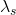is the source wavelength, and v is the relative velocity of the source to the observer.

### Conceptual Questions

Explain the meaning of the terms “red shift” and “blue shift” as they relate to the relativistic Doppler effect.

What happens to the relativistic Doppler effect when relative velocity is zero? Is this the expected result?

There is no measured change in wavelength or frequency in this case. The relativistic Doppler effect depends only on the relative velocity of the source and the observer, not any speed relative to a medium for the light waves.

Is the relativistic Doppler effect consistent with the classical Doppler effect in the respect thatis larger for motion away?

All galaxies farther away than about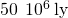exhibit a red shift in their emitted light that is proportional to distance, with those farther and farther away having progressively greater red shifts. What does this imply, assuming that the only source of red shift is relative motion?

It shows that the stars are getting more distant from Earth, that the universe is expanding, and doing so at an accelerating rate, with greater velocity for more distant stars.]

### Problems

A highway patrol officer uses a device that measures the speed of vehicles by bouncing radar off them and measuring the Doppler shift. The outgoing radar has a frequency of 100 GHz and the returning echo has a frequency 15.0 kHz higher. What is the velocity of the vehicle? Note that there are two Doppler shifts in echoes. Be certain not to round off until the end of the problem, because the effect is small.